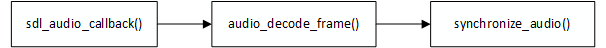# FFplay音频同步分析—ffplay.c源码分析

ffplay -sync video -i juren-30s.mp4synchronize_audio() 函数就是用来实现音频同步逻辑的af->frame->nb_samples 代表 AVFrame 原来的样本数，而 wanted_nb_samples 代表 AVFrame 调整之后的样本数，通常会增加样本数或者减少样本数。

synchronize_audio() 函数的重点代码如下：synchronize_audio() 函数的音频同步算法也是类似的套路，如果超过 AV_NOSYNC_THRESHOLD（10s） 就不处理，跟之前《FFplay视频同步分析》里面的视频同步逻辑一样。

synchronize_audio() 函数的音频同步算法有点复杂，主要涉及到两个数学概念。

1，加权平均数

2，等比数列

加权平均数 = 加权总和 / 权数总和


1，AV_NOSYNC_THRESHOLD，这个宏是 10，单位是秒，所以如果差异太大，超过10s，直接不调整样本数，跟之前《FFplay视频同步分析》里面的视频同步逻辑一样，都是超过 10s 不进行同步。

2，AUDIO_DIFF_AVG_NB，这是一个数量值，值为 20，这个其实是取 20 次以上的音视频差异来计算 加权平均数。越前面的差异时间权重越大。例如 第 21 次的音视频时间差异 的权重大于 第 20 次的权重，第 20 次的权重大于 第 19 次的权重。

ffplay.c 里面，加权平均数就是 avg_diff 变量，下面会讲到。

3，is->audio_diff_avg_coef，这个变量实际上是 等比数列 里面的 公比q ，代码如下：

is->audio_diff_avg_coef  = exp(log(0.01) / AUDIO_DIFF_AVG_NB);


4，is->audio_diff_cum，这是20次音视频差异的加权总和，代码如下：

is->audio_diff_cum = diff + is->audio_diff_avg_coef * is->audio_diff_cum;


a_diff 为第一次差异，b_diff 为第二次差异，c_diff 为第三次差异。

is->audio_diff_cum = c_diff * 1 + ((b_diff + (a_diff * 0.79432) ) * 0.79432)


is->audio_diff_cum =
a * (0.79 ^ 23) +
b * (0.79 ^ 22) +
c * (0.79 ^ 21) +
d * (0.79 ^ 20) +
....
z * (0.79 ^ 0)


$w = (0.79 ^ {23}) + (0.79 ^ {22}) + (0.79 ^ {21}) + ... + (0.79 ^ {0})$a1 是首项，所以 a1 等于 1。而 q^n 是 0.79 乘以 20 次以上的值，可以忽略不计。 $a1 = 0.79^0 = 1$

$q^n = 0.79^{20} = 0.01$

$avg\_diff = audio\_diff\_cum \div ( 1 \div (1-0.79))$

$avg\_diff = audio\_diff\_cum \times (1-0.79)$

avg_diff = is->audio_diff_cum * (1.0 - is->audio_diff_avg_coef);


avg_diff 就是20 次以上的音视频差异的加权平均数。

6，is->audio_diff_threshold，音频同步阈值，音视频不同步的程度超过这个值，就会进行干预。计算方式如下：

/* since we do not have a precise anough audio FIFO fullness,
we correct audio sync only if larger than this threshold */
is->audio_diff_threshold = (double)(is->audio_hw_buf_size) / is->audio_tgt.bytes_per_sec;


if (fabs(avg_diff) >= is->audio_diff_threshold) {
wanted_nb_samples = nb_samples + (int)(diff * is->audio_src.freq);
min_nb_samples = ((nb_samples * (100 - SAMPLE_CORRECTION_PERCENT_MAX) / 100));
max_nb_samples = ((nb_samples * (100 + SAMPLE_CORRECTION_PERCENT_MAX) / 100));
wanted_nb_samples = av_clip(wanted_nb_samples, min_nb_samples, max_nb_samples);
}


SAMPLE_CORRECTION_PERCENT_MAX 宏的值为 10，所以替换之后如下：

if (fabs(avg_diff) >= is->audio_diff_threshold) {
wanted_nb_samples = nb_samples + (int)(diff * is->audio_src.freq);
min_nb_samples = ((nb_samples * 0.9);
max_nb_samples = ((nb_samples * 1.1);
wanted_nb_samples = av_clip(wanted_nb_samples, min_nb_samples, max_nb_samples);
}


if (wanted_nb_samples != af->frame->nb_samples) {
if (swr_set_compensation(is->swr_ctx,
(wanted_nb_samples - af->frame->nb_samples) * is->audio_tgt.freq / af->frame->sample_rate,
wanted_nb_samples * is->audio_tgt.freq / af->frame->sample_rate) < 0) {
av_log(NULL, AV_LOG_ERROR, "swr_set_compensation() failed\n");
return -1;
}
}